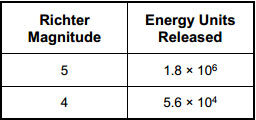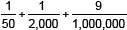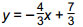# General Curriculum Study Guide

## Mathematics SubtestSample Multiple-Choice Questions

#### Numbers and Operations

##### Objective 0016 Understand the number system and the concept of place value.

1. Use the information below to complete the exercise that follows.The Richter scale is a system used by geologists for measuring the severity of earthquakes. What is the difference, expressed to two significant digits, between the amount of energy released by an earthquake of Richter magnitude 5 and an earthquake of magnitude 4?

1. 3.8 × 102 units
2. 1.7 × 105 units
3. 3.8 × 105 units
4. 1.7 × 106 units
Correct Response: D.

##### Objective 0017 Understand integers, fractions, decimals, percents, and mixed numbers.

2. Which of the following decimals is equal to?

1. 0.0020509
2. 0.002509
3. 0.0205009
4. 0.020509
Correct Response: D.

#### Functions and Algebra

##### Objective 0022 Understand linear functions and linear equations.

3. Use the graph below to answer the question that follows.The graph of which of the following equations is a line parallel to the line graphed above?

1.2.3.4.Correct Response: A.

##### Objective 0024 Understand and apply concepts of geometry.

4. Use the diagram below to answer the question that follows.The polygon shown above is regular. What is the sum of angles x and y?

1. 108°
2. 120°
3. 132°
4. 144°
Correct Response: D.

#### Statistics and Probablility

##### Objective 0026 Understand and apply basic concepts of probability.

5. A part-time salesperson works 2 days per week at a store that is open Monday through Friday. If the workdays are randomly assigned, what is the probability that the salesperson will work on Monday and Tuesday?

1. 1/20
2. 1/10
3. 2/5
4. 2/3